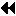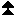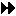MPI library implementation

## MPI library implementationUp: Examples Next: Systems with weak symbols Previous: Profiler implementation

On a Unix system, in which the MPI library is implemented in C, then there are various possible options, of which two of the most obvious are presented here. Which is better depends on whether the linker and compiler support weak symbols.Up: Examples Next: Systems with weak symbols Previous: Profiler implementation

### Systems with weak symbolsUp: MPI library implementation Next: Systems without weak symbols Previous: MPI library implementation

If the compiler and linker support weak external symbols (e.g. Solaris 2.x, other system V.4 machines), then only a single library is required through the use of #pragma weak thus

```#pragma weak MPI_Example = PMPI_Example

int PMPI_Example(/* appropriate args */)
{
/* Useful content */
}
```
The effect of this #pragma is to define the external symbol MPI_Example as a weak definition. This means that the linker will not complain if there is another definition of the symbol (for instance in the profiling library), however if no other definition exists, then the linker will use the weak definition.Up: MPI library implementation Next: Systems without weak symbols Previous: MPI library implementation

### Systems without weak symbolsUp: MPI library implementation Next: Complications Previous: Systems with weak symbols

In the absence of weak symbols then one possible solution would be to use the C macro pre-processor thus

```#ifdef PROFILELIB
#    ifdef __STDC__
#        define FUNCTION(name) P##name
#    else
#        define FUNCTION(name) P/**/name
#    endif
#else
#    define FUNCTION(name) name
#endif
```
Each of the user visible functions in the library would then be declared thus

```int FUNCTION(MPI_Example)(/* appropriate args */)
{
/* Useful content */
}
```
The same source file can then be compiled to produce both versions of the library, depending on the state of the PROFILELIB macro symbol.

It is required that the standard MPI library be built in such a way that the inclusion of MPI functions can be achieved one at a time. This is a somewhat unpleasant requirement, since it may mean that each external function has to be compiled from a separate file. However this is necessary so that the author of the profiling library need only define those MPI functions which she wishes to intercept, references to any others being fulfilled by the normal MPI library. Therefore the link step can look something like this

```% cc ... -lmyprof -lpmpi -lmpi
```
Here libmyprof.a contains the profiler functions which intercept some of the MPI functions. libpmpi.a contains the ``name shifted'' MPI functions, and libmpi.a contains the normal definitions of the MPI functions.Up: MPI library implementation Next: Complications Previous: Systems with weak symbols